For this initial project, I was inspired by the overwhelming amount of nail polish I own and cannot seem to organize.I used really basic functions (mostly translate) to design this object but I am overall proud of myself and am happy with the finished design.  I did not use anyone else’s code to complete this.

I would like to go back to this and figure out how to reduce the amount of code I used.  I remember during one of our online meetings we discussed defining modules, and I think that would help here.  I am still uncomfortable with a lot of the code in OpenSCAD, but I am slowly getting better.

```//nail polish holder//
difference() {
// original
cube (size = [42,42,30]);
// hole
translate ([2,2,2]) {
cube (size = [38,38,32]);
}
}
translate([0,42,0]) {
difference() {
// original
cube (size = [42,42,30]);
// hole
translate ([2,2,2]) {
cube (size = [38,38,32]);
}
}
}
translate([0,84,0]){
difference() {
// original
cube (size = [42,42,30]);
// hole
translate ([2,2,2]) {
cube (size = [38,38,32]);
}
}
}
translate([0,126,0]){
difference() {
// original
cube (size = [42,42,30]);
// hole
translate ([2,2,2]) {
cube (size = [38,38,32]);
}
}
}
translate([0,168,0]){
difference() {
// original
cube (size = [42,42,30]);
// hole
translate ([2,2,2]) {
cube (size = [38,38,32]);
}
}
}
translate([42,0,0]){
difference() {
// original
cube (size = [42,42,30]);
// hole
translate ([2,2,2]) {
cube (size = [38,38,32]);
}
}
}
translate([84,0,0]){
difference() {
// original
cube (size = [42,42,30]);
// hole
translate ([2,2,2]) {
cube (size = [38,38,32]);
}
}
}
translate([126,0,0]){
difference() {
// original
cube (size = [42,42,30]);
// hole
translate ([2,2,2]) {
cube (size = [38,38,32]);
}
}
}
translate([168,0,0]){
difference() {
// original
cube (size = [42,42,30]);
// hole
translate ([2,2,2]) {
cube (size = [38,38,32]);
}
}
}
translate([210,0,0]){
difference() {
// original
cube (size = [42,42,30]);
// hole
translate ([2,2,2]) {
cube (size = [38,38,32]);
}
}
}
translate([42,42,0]){
difference() {
// original
cube (size = [42,42,30]);
// hole
translate ([2,2,2]) {
cube (size = [38,38,32]);
}
}
}
translate([42,84,0]){
difference() {
// original
cube (size = [42,42,30]);
// hole
translate ([2,2,2]) {
cube (size = [38,38,32]);
}
}
}
translate([42,126,0]){
difference() {
// original
cube (size = [42,42,30]);
// hole
translate ([2,2,2]) {
cube (size = [38,38,32]);
}
}
}
translate([42,168,0]){
difference() {
// original
cube (size = [42,42,30]);
// hole
translate ([2,2,2]) {
cube (size = [38,38,32]);
}
}
}
translate([84,42,0]){
difference() {
// original
cube (size = [42,42,30]);
// hole
translate ([2,2,2]) {
cube (size = [38,38,32]);
}
}
}
translate([84,84,0]){
difference() {
// original
cube (size = [42,42,30]);
// hole
translate ([2,2,2]) {
cube (size = [38,38,32]);
}
}
}
translate([84,126,0]){
difference() {
// original
cube (size = [42,42,30]);
// hole
translate ([2,2,2]) {
cube (size = [38,38,32]);
}
}
}
translate([84,168,0]){
difference() {
// original
cube (size = [42,42,30]);
// hole
translate ([2,2,2]) {
cube (size = [38,38,32]);
}
}
}
translate([126,42,0]){
difference() {
// original
cube (size = [42,42,30]);
// hole
translate ([2,2,2]) {
cube (size = [38,38,32]);
}
}
}
translate([126,84,0]){
difference() {
// original
cube (size = [42,42,30]);
// hole
translate ([2,2,2]) {
cube (size = [38,38,32]);
}
}
}
translate([126,126,0]){
difference() {
// original
cube (size = [42,42,30]);
// hole
translate ([2,2,2]) {
cube (size = [38,38,32]);
}
}
}
translate([126,168,0]){
difference() {
// original
cube (size = [42,42,30]);
// hole
translate ([2,2,2]) {
cube (size = [38,38,32]);
}
}
}
translate([168,42,0]){
difference() {
// original
cube (size = [42,42,30]);
// hole
translate ([2,2,2]) {
cube (size = [38,38,32]);
}
}
}
translate([168,84,0]){
difference() {
// original
cube (size = [42,42,30]);
// hole
translate ([2,2,2]) {
cube (size = [38,38,32]);
}
}
}
translate([168,126,0]){
difference() {
// original
cube (size = [42,42,30]);
// hole
translate ([2,2,2]) {
cube (size = [38,38,32]);
}
}
}
translate([168,168,0]){
difference() {
// original
cube (size = [42,42,30]);
// hole
translate ([2,2,2]) {
cube (size = [38,38,32]);
}
}
}
translate([210,42,0]){
difference() {
// original
cube (size = [42,42,30]);
// hole
translate ([2,2,2]) {
cube (size = [38,38,32]);
}
}
}
translate([210,84,0]){
difference() {
// original
cube (size = [42,42,30]);
// hole
translate ([2,2,2]) {
cube (size = [38,38,32]);
}
}
}
translate([210,126,0]){
difference() {
// original
cube (size = [42,42,30]);
// hole
translate ([2,2,2]) {
cube (size = [38,38,32]);
}
}
}
translate([210,168,0]){
difference() {
// original
cube (size = [42,42,30]);
// hole
translate ([2,2,2]) {
cube (size = [38,38,32]);
}
}
}
//side file holder
translate([252,0,0]){
difference() {
// file holder
cube (size = [42,210,10]);
// hole
translate ([2,2,2]) {
cube (size = [38,206,11]);
}
}
}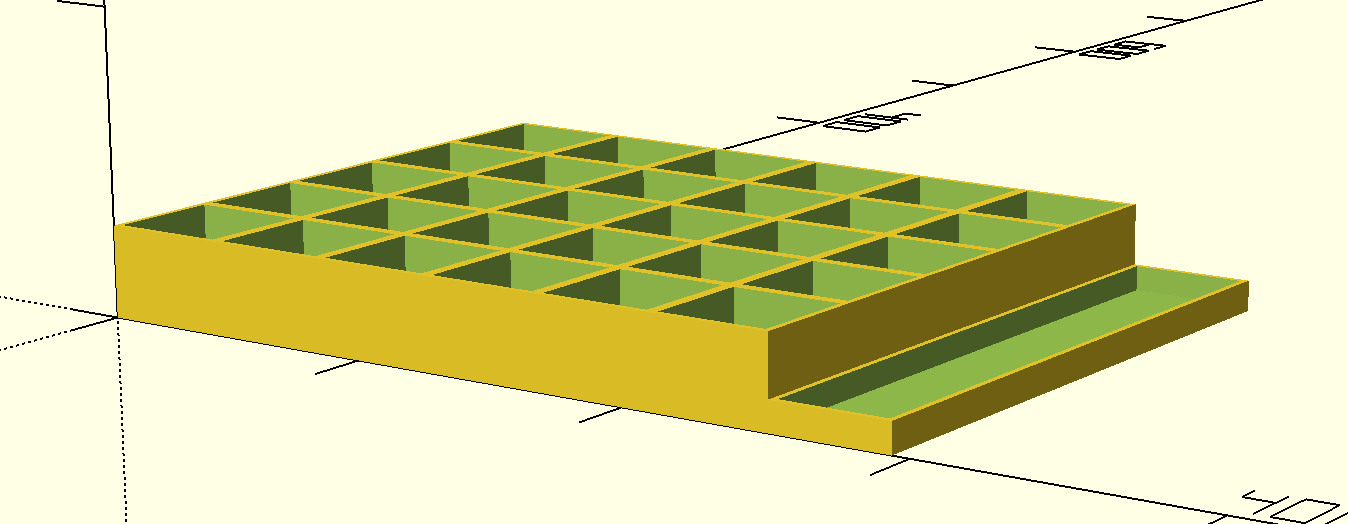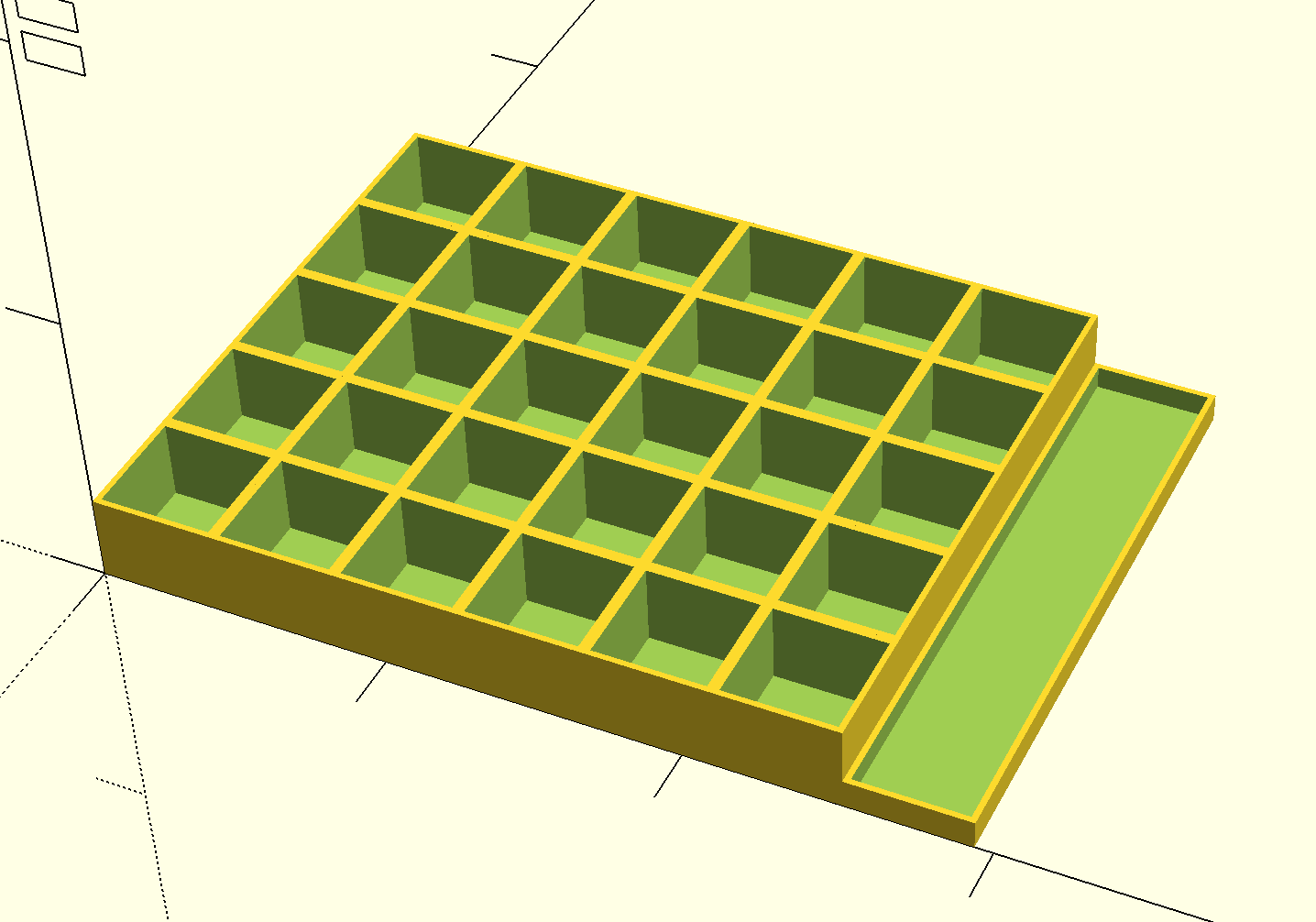```

For this week’s design, I created an all-in-one docking station for my phone, smartwatch, wireless headphones, and glasses.  I was inspired by docking stations that I have seen online but I think are too expensive.  I already have a small unit that contains my headphones and watch and I wanted a design that worked around the existing unit so that I can avoid being wasteful.

I used the Minkowski function for the first time in this design to round out the corners of the base.  I referenced our past class discussions and the OpenSCAD cheat sheet in order to accomplish this.  I also used the hull function to create the spaces for my glass and the watch/headphone unit.  The hull function essentially creates two circles connected by an overlapping rectangle which I thought was perfect for what I wanted.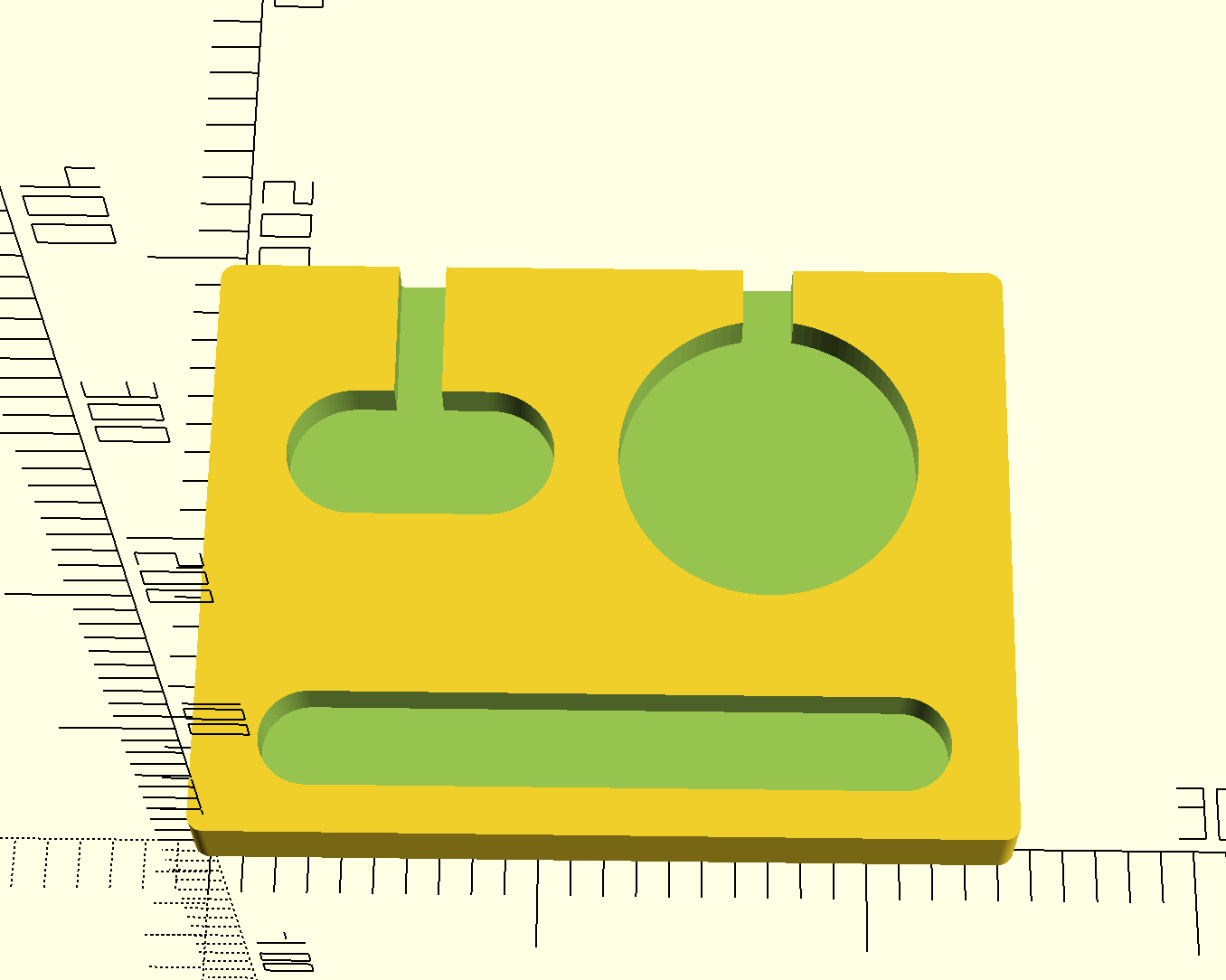If I were to make another iteration of this, I would like to figure out how to round out the edges of the places where I used the difference function. I think it would make the overall piece look more finished.  I could even learn how to carve in initials or words to make the piece more personalized.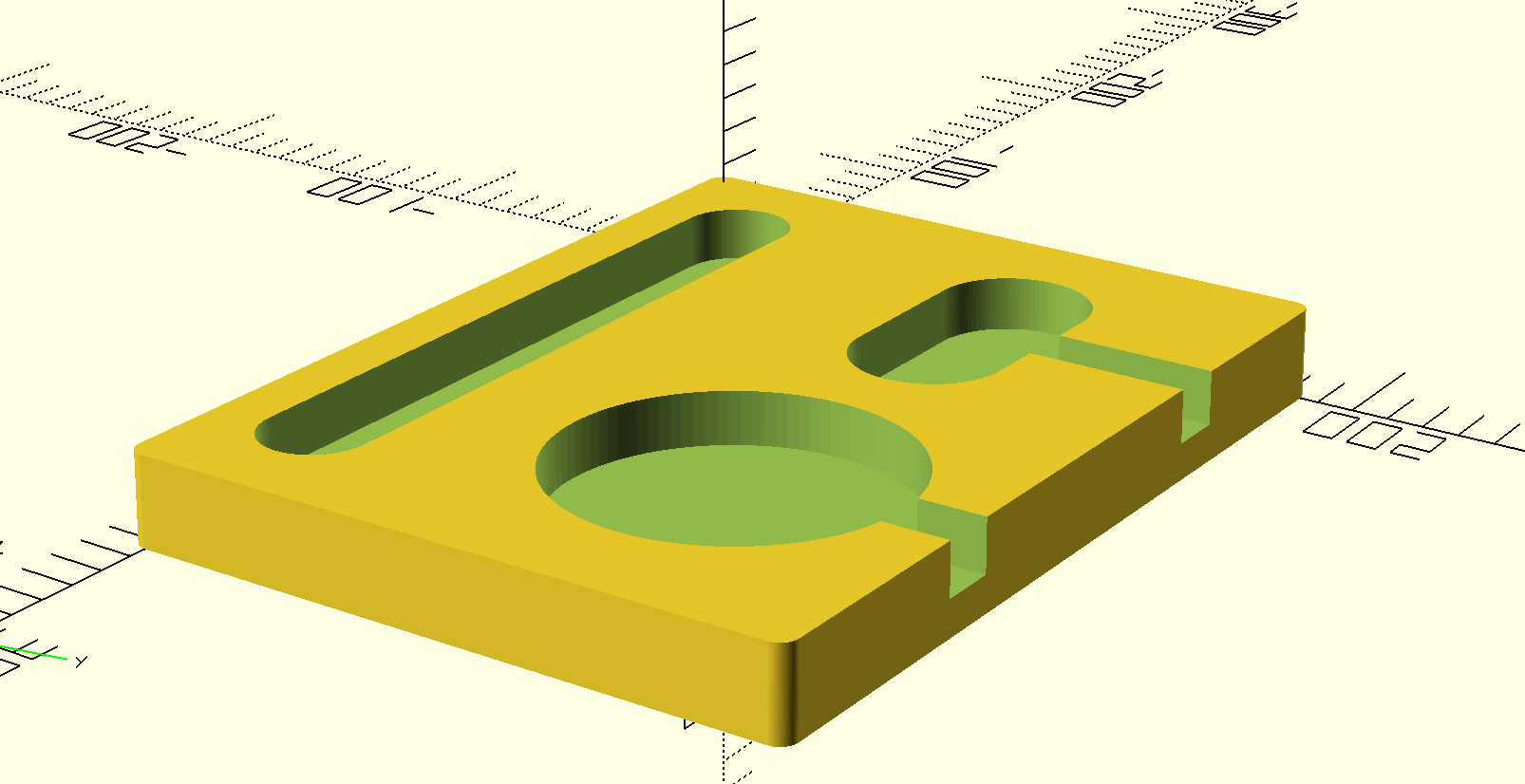*side note* my boyfriend looked at this and said this design looks like a stressed robot.

```//docking station v1//
\$fn=100;

difference(){
//base//
minkowski(){
cube([240,180,24]);
cylinder(d1=10, d2=10 , h=.5);

}
//watch//
translate([40,120,10]){
hull() {
linear_extrude(15)
linear_extrude(15)
}
}
translate([52,140,10]){
cube([15,46,15]);
}
//glasses//
translate([30,25,10]){
hull() {
linear_extrude(15)
circle(15);
linear_extrude(15)
translate([180, 0, 0])
circle(15);
}
}

//phone//
translate([170,120,10]){
cylinder(d1=94, d2=94, h=40);
}
translate([162,140,10]){
cube([16,51,15]);
}
}```## Iteration

I decided to do an iteration on the original nail polish holder I designed.  Last week’s docking station felt mostly complete to me and I felt I could add more to week one’s project.  What I did not realize was that I lost the code from week 1 and the preformatted code in the above post saved as one continuous line of data.  I spent far too long going back and having to figure out where to separate the different lines of code.  Nevertheless, I did manage to add a few new things, albeit not as much as I originally planned.

I originally wanted to round out some of the edges to make the overall piece look a bit softer, but I did not get to that in time.  I did add holders for cotton rounds and q-tips on the left side of the design.  I wanted to have small cutouts on the longest side where the q-tips could roll out kind of like those toothpick dispensers you see in some restaurants, but I could not figure it out.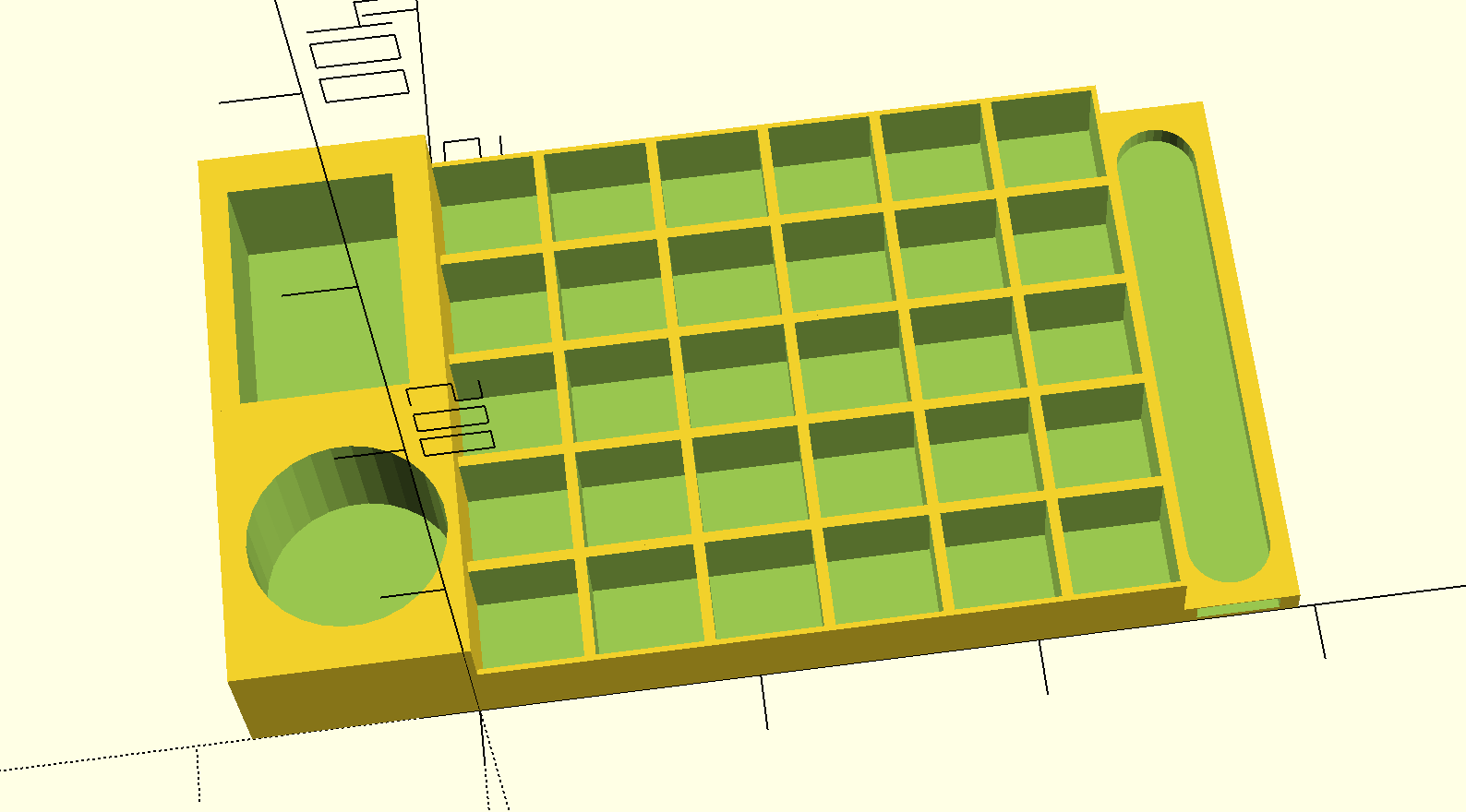I used the hull function that I learned last week to make the //side file holder// section less clunky.

Overall, I am happy with how this design has turned out, even though I was unable to get everything I had planned.

Here is the new code added this week:

```//side file holder
translate([252,0,0]){
difference() {
// file holder
cube (size = [42,210,10]);
// hole
translate ([20,0,2]) {
hull() {
linear_extrude(7)
circle(15);
linear_extrude(15)
translate([0,185,0])
circle(15);
}
}
}
}

//cotton rounds
translate([-80.5,0,0]){
difference(){
//base
cube (size = [83,105,50]);
//hole
translate ([44,50,5]) {
cylinder ( h= 50, r1= 35, r2=35);
}
}
}
//q tips
translate([-80.5,105,0]){
difference(){
//base
cube (size = [83,105,50]);
//hole
translate([10,2,5]){
cube (size = [60,88,65]);
}
}
}

```

## Week Five: Randomness

I was honestly terrified and not excited about implementing randomness into my design.  It looked like something that was going to be way over my head and my abilities, but I managed to work something out.

Before embarking on randomness, I figured out how to create a module of each of the nail polish holders in my design.  This cleaned up my overall code tremendously and was much easier than I anticipated.  I wish I had figured it out sooner as it would have saved me a lot of time last week.  I initially did this because I wanted to randomize the heights and occurrences of each of the holders.  That did not happen.

I did edit the code given to us to use the polish module instead of the cube.  I also changed the position of each random cube to be spaced apart in a way that they are still touching (if present).  The code does make random cubes, but they are still at true center, even when I deleted that command and tried to replace it.  I also do not know how to randomize the overall positions.  Ideally, I would have wanted for the random code to draw from the existing 30 positions of nail polish holders and change the height and occurrence of them.  After playing around with it I gave up after a lot of frustration and googling.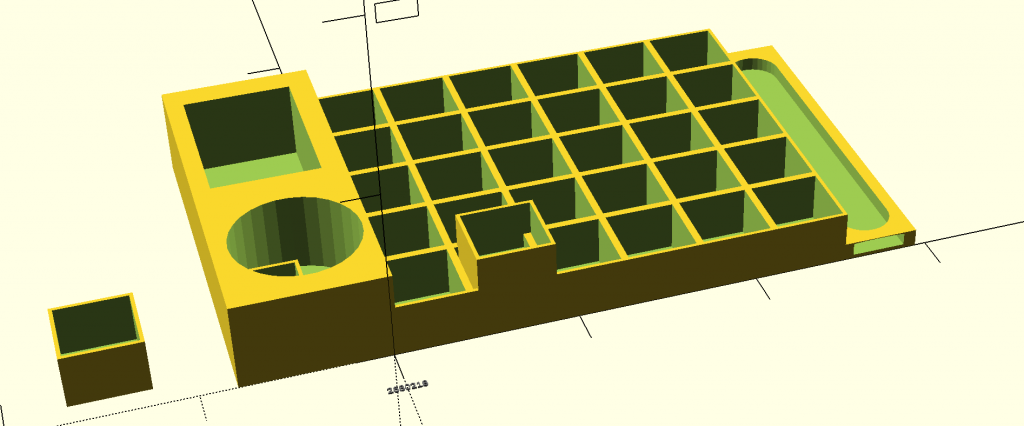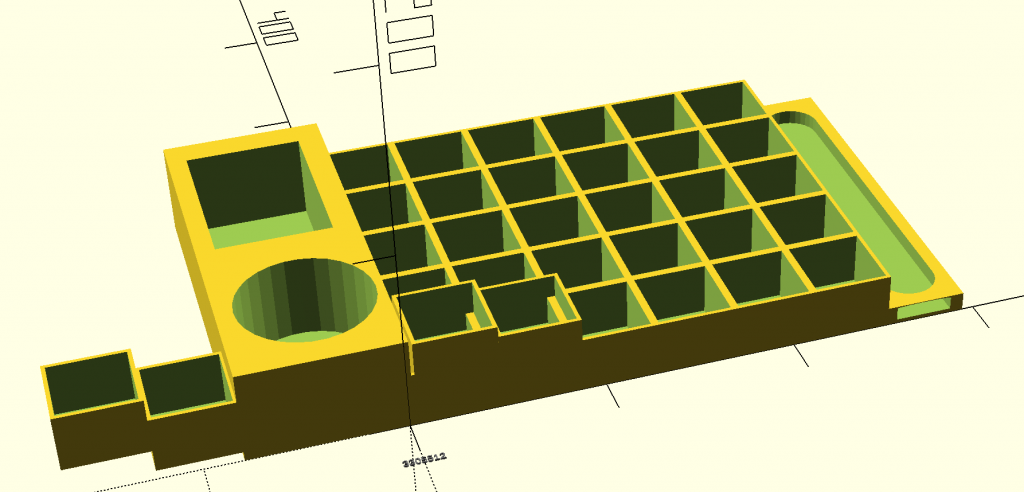I did ask my boyfriend, who is a software developer by trade, to help me with this and his only response was “This is not the kind of coding I do.”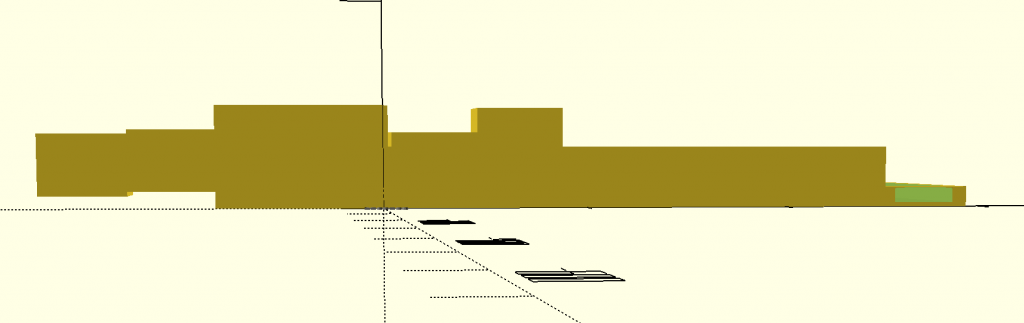```///////////////////////////////////////////////////////////////////
// CONTROLLING THE RANDOMNESS

// controls scaling of elements in random subsequence1
// number from 0 (min allowable) to 10 (max allowable)
style1 = 5;

// controls likelihood of true/false in random subsequence2
// number from 0 (always false) to 10 (always true)
style2 = 4;

///////////////////////////////////////////////////////////////////
// RANDOM SEED

// LET OPENSCAD CHOOSE A RANDOM SEED
random_seed = floor(rands(1,9999999,1));

// OR TYPE IN A SEED MANUALLY
// Overrides the slider seed unless set to 0
custom_seed = 0;

// set the random seed
seed = (custom_seed==0) ? random_seed : custom_seed;

// Show random seed? (it won't print even if you say yes)
show_seed = "yes"; //[yes:Yes,no:No]

// Create a string to output the random seed
// thank you laird for showing me how to do this with your code
// from http://www.thingiverse.com/thing:188481
labelString=str(floor(seed/1000000)%10, floor(seed/100000)%10,
floor(seed/10000)%10, floor(seed/1000)%10,
floor(seed/100)%10, floor(seed/10)%10,
floor(seed/1)%10);

// Display the random seed in the render (F5 only)
if(show_seed=="yes"){
translate([0,-30,0])
color("gray")
%linear_extrude(height=1)
text(labelString,size=4,halign="center");
}

// Output the random seed in the log
echo(labelString);

///////////////////////////////////////////////////////////////////
// RANDOM SEQUENCES

// construct the main random sequence
// a list of random real numbers in [0,10] based on the chosen seed
maxSteps=30*1;
random = rands(0,6,maxSteps,seed);
echo(random);

// subsequence of 10 items from the main sequence
// weighted by style1
subrandom1 = [for(i=[0:6-1]) random[i]*style1];
echo(subrandom1);

// subsequence of another 10 items from the main sequence
// output as true/false verdicts by comparison to style2
subrandom2 = [for(i=[10:10+10-1]) random[i]<=style2];
echo(subrandom2);

///////////////////////////////////////////////////

for (i=[0:6-1]){
if (subrandom2[i]==true){
translate([(i-4)*(42)+100/24,0,5+subrandom1[i]/2])
polish([5,5,subrandom1[i]]);
}
}

///////////////////////////////////////////////////////
//nail polish holder//
module polish()
{
difference() {
// original
cube (size = [42,42,30]);
// hole
translate ([2,2,2]) {
cube (size = [38,38,32]);
}
}
}
polish();

translate([0,42,0]) {
polish();
}
translate([0,84,0]){
polish();
}
translate([0,126,0]){
polish();
}
translate([0,168,0]){
polish();
}
translate([42,0,0]){
polish();
}
translate([84,0,0]){
polish();
}
translate([126,0,0]){
polish();
}
translate([168,0,0]){
polish();
}
translate([210,0,0]){
polish();
}
translate([42,42,0]){
polish();
}
translate([42,84,0]){
polish();
}
translate([42,126,0]){
polish();
}
translate([42,168,0]){
polish();
}
translate([84,42,0]){
polish();
}
translate([84,84,0]){
polish();
}
translate([84,126,0]){
polish();
}
translate([84,168,0]){
polish();
}
translate([126,42,0]){
polish();
}
translate([126,84,0]){
polish();
}
translate([126,126,0]){
polish();
}
translate([126,168,0]){
polish();
}
translate([168,42,0]){
polish();
}
translate([168,84,0]){
polish();
}

translate([168,126,0]){
polish();
}
translate([168,168,0]){
polish();
}

translate([210,42,0]){
polish();
}
translate([210,84,0]){
polish();
}
translate([210,126,0]){
polish();
}
translate([210,168,0]){
polish();
}
///////////////////////////////////////////////////
//side file holder
translate([252,0,0]){
difference() {
// file holder
cube (size = [42,210,10]);
// hole
translate ([20,0,2]) {
hull() {
linear_extrude(7)
circle(15);
linear_extrude(15)
translate([0,185,0])
circle(15);
}
}
}
}

//cotton rounds
translate([-80.5,0,0]){
difference(){
//base
cube (size = [83,105,50]);
//hole
translate ([44,50,5]) {
cylinder ( h= 50, r1= 35, r2=35);
}
}
}
//q tips
translate([-80.5,105,0]){
difference(){
//base
cube (size = [83,105,50]);
//hole
translate([10,2,5]){
cube (size = [60,88,65]);
}
}
}

```

##Final Iteration

For the final iteration, I managed to figure out how to randomize the appearance (true/false) of the individual boxes.  I took the given random parameters code and modified it a bit by only having one style and one subrandom command.  This proved to be much more difficult than I anticipated but through some assistance, I have come up with an outcome that I am happy with!

Even though I know this code is fairly simple in the grand scheme of things, it is much more complex than I ever thought I would be able to accomplish.  I did not take this class expecting to learn coding, but I am happy with the progress I have made over the last few weeks.I am able to manipulate the code in a way where I can have all 30 of the cubes present, none at all, or a randomized number.  I don’t know how practical this build would be without having all of the cubes present, but it is neat to play around with.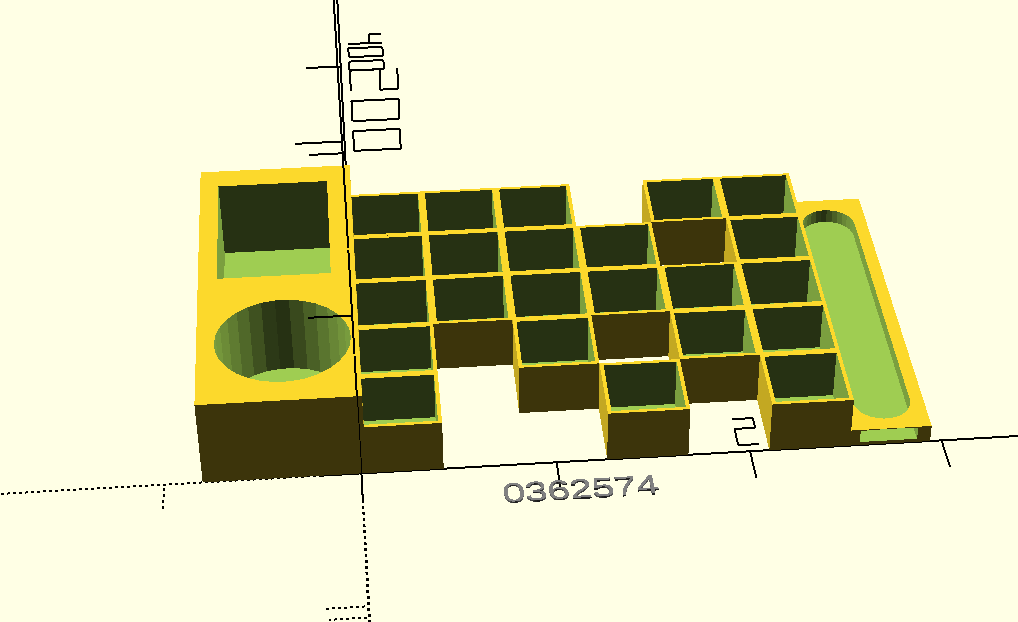```//////////////////////////////////////////////////////////
//////////////////////////////////////////////////////////////
// RANDOM SEED```
```// LET OPENSCAD CHOOSE A RANDOM SEED
random_seed = floor(rands(1,9999999,1));```
`custom_seed = 0;`
`seed = (custom_seed==0) ? random_seed : custom_seed;`
`show_seed = "yes"; //[yes:Yes,no:No]`
```labelString=str(floor(seed/1000000)%10, floor(seed/100000)%10,
floor(seed/10000)%10, floor(seed/1000)%10,
floor(seed/100)%10, floor(seed/10)%10,
floor(seed/1)%10);```
```// Display the random seed in the render (F5 only)
if(show_seed=="yes"){
translate([110,-30,0])
color("gray")
%linear_extrude(height=1)
text(labelString,size=15,halign="center");
}```
```// Output the random seed in the log
echo(labelString);```
```// Output the random seed in the log
echo(labelString);```
`//////////////////////////////////////////////////////////////`
```//  RANDOM SEQUENCES
// style determines the likelihood of the box appearing (true/false) closer to 6 = more boxes, closer to 0 = less boxes
style= 4.8;```
```// list1 contains all of my squares
list1 = [ for (i = [0 : 42 : 210]) for (j = [0 : 42 : 168]) [i,j,0]];
echo(list1);
echo(list1.size);
// construct the main random sequence
// a list of 30 random real numbers in [0,6] based on the chosen seed
maxSteps=30*1;
random = rands(0,6,maxSteps,seed);
echo(random);```
```// subsequence of another 10 items from the main sequence
// output as true/false verdicts by comparison to style
subrandom = [for(i=[0:30-1]) random[i]<=style];
echo(subrandom);```
```///////////////////////////////////////////////////////////////
for (i = [0:30-1]){
if (subrandom[i]==true) {
translate(list1[i]) polish(30);
}
}```
```///////////////////////////////////////////////////////
//nail polish holder//
module polish(height)
{
difference() {
// original
cube (size = [42,42,height]);
// hole
translate ([2,2,2]) {
cube (size = [38,38,height+2]);
}
}
}

```
```///////////////////////////////////////////////////
//side file holder
translate([252,0,0]){
difference() {
// file holder
cube (size = [42,210,10]);
// hole
translate ([20,0,2]) {
hull() {
linear_extrude(7)
circle(15);
linear_extrude(15)
translate([0,185,0])
circle(15);
}
}
}
}

//cotton rounds
translate([-80.5,0,0]){
difference(){
//base
cube (size = [83,105,50]);
//hole
translate ([44,50,5]) {
cylinder ( h= 50, r1= 35, r2=35);
}
}
}
//q tips
translate([-80.5,105,0]){
difference(){
//base
cube (size = [83,105,50]);
//hole
translate([10,2,5]){
cube (size = [60,88,65]);
}
}
}

```